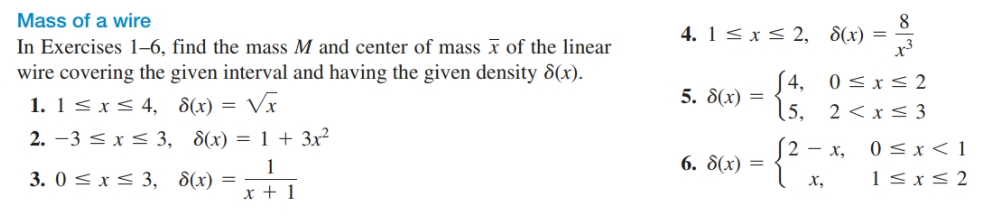# Mass of a wire In Exercises 1–6, find the mass M and center of mass x of the linear wire covering the given interval and having the given density 8(x). 1. 1< xS 4, 8(x) = Vx 2. -3 < x < 3, 8(x) = 1 + 3x² 3. 0 < x< 3, 8(x) = 4. 1< x< 2, 8(x) x3 = S4, 0

Questionhelp_outlineImage TranscriptioncloseMass of a wire In Exercises 1–6, find the mass M and center of mass x of the linear wire covering the given interval and having the given density 8(x). 1. 1< xS 4, 8(x) = Vx 2. -3 < x < 3, 8(x) = 1 + 3x² 3. 0 < x< 3, 8(x) = 4. 1< x< 2, 8(x) x3 = S4, 0